# One Way ANOVA using SPSS

Learn to Run, Interpret, and Report One WAY ANOVA using SPSS

## Concept of One Way ANOVA

Where One-Sample t-test is used to compare mean of sample against some standard value or mean of the population, Independent Samples t-test is used to compare if there are differences in the sample mean for two different groups. What if you have three different groups and you are required to check the differences across the three groups.

One-way ANOVA is also referred to as One-way between-groups ANOVA because you will have one independent (grouping) variable with three or more levels (groups) and one dependent continuous variable. In simplest of words One-Way ANOVA is used to compares scores of 3 or more than 3 groups.

### Examples of One Way ANOVA

Following are few scenarios in which you would consider using One-Way ANOVA

• An Economist would like to know if there are differences in income of Lecturers across the cities of London, Paris, and Islamabad.
• Is there a difference in optimism levels across three age levels?
• A manager wishes to evaluate if staff satisfaction scores differs across permanent, Part time, and casual staff

In each of the above-mentioned situation there is one dependent variable and an independent variable that has different categories. One-Way ANOVA allows checking for differences across the different categories for the dependent variable. It is called analysis of variance because it compares the variance (variability in scores) between the different groups (believed to be due to the independent variable).

### Requirements

Information Required:

• A continuous dependent variable, in this case Organizational Commitment
• A Categorical Variable having more than two categories, in this case it is Management Levels (Junior, Middle, and Senior)

### How to Run One Way ANOVA in SPSS

Step 1: Go to Analysis -> Compare Means -> One-Way ANOVA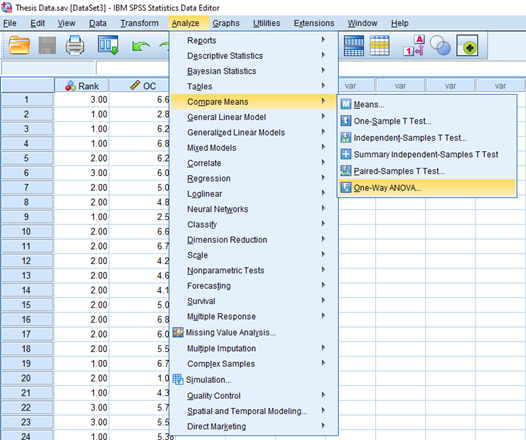Step 2: From the Dialog Box, Add the Criterion (Dependent) Variable into the Dependent List, and Categorical Variable into the Factor List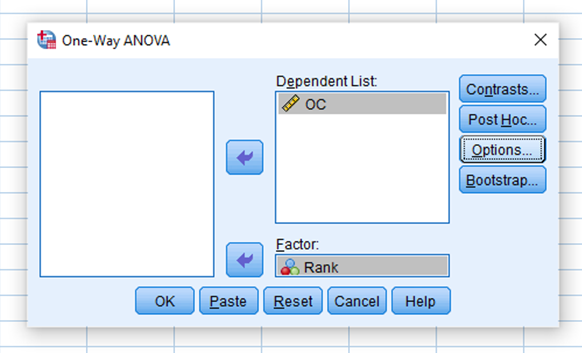Step 3: Select Descriptive and Homogeneity of variance test and Press Continue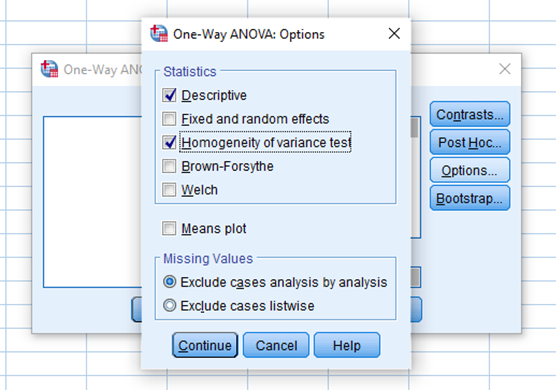Step 4: Now Press OK

### Interpreting One Way ANOVA Results

SPSS Output Window will present the following results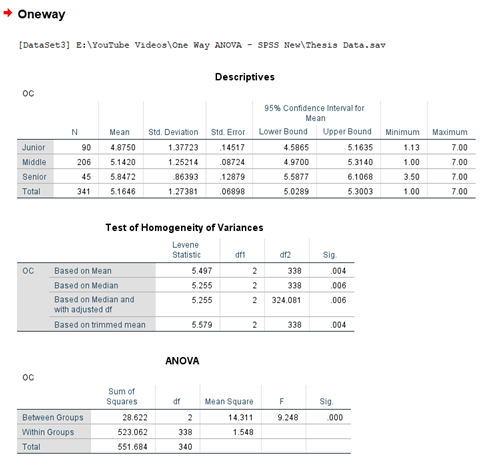The results show three tables,

Descriptives: Shows the descriptive statistics for each of the category on the dependent variable. For instance, in case of Junior Level Employees, the average Organizational Commitment was 4.87.

Test of Homogeneity of Variances: The next table of Test of Homogeneity of Variances shows you the results of Levene’s test; the purpose is similar to Levene’s test of independent sample t-test. In that you check for variance between two groups, however in this case the variances are test for more than 2 groups. Since the value of Sig. for Levene’s test is less than .05 we would infer that there are significant differences in the variation of score across the age groups. Keep the value in mind you will use this value in Post Hoc Analysis to be discussed later in the chapter.

ANOVA: ANOVA table is of main interest. You are actually interest in knowing if there are any significant differences on the dependent variable (Organizational Commitment) across the groups. Now if the significance value if less than .05 you would infer that there are significant differences across the groups. In this particular case the Sig value is .000 (p < .01) thus there are significant differences in Organizational Commitment across the different Management Levels.

But the question is since we have 3 groups, and there are significant differences across these 3 groups.  The problem now is that if someone asks you which group is different from which group, the results from the ANOVA table do not let the researcher know the significant of differences between two groups. The statistical significance of the differences between each pair of groups is provided through Multiple Comparisons, for this you would have to run post-hoc tests.

#### Run Post-Hoc Analysis:

Select Post Hoc Button from the One-Way ANOVA Dialog Box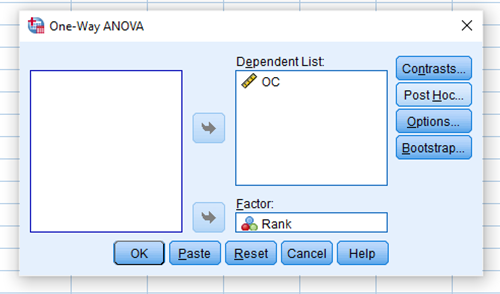In this scenario, since Homogeneity of Variances was not assumed, So We select Dunnett’s T3 from Equal Variances Not Assumed group box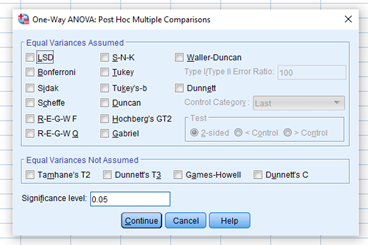Apart from all the tables that we discussed earlier, One-Way ANOVA results display a new table of Multiple Comparisons; this table would guide you if specific groups differ significantly from each other. You should only run the Post-Hoc analysis only when there are significant overall differences as shown in the ANOVA table.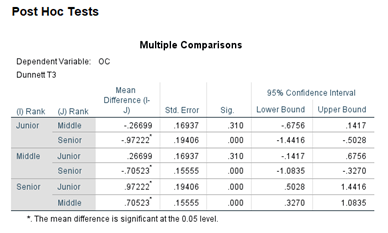Looking at the Multiple Comparisons table, the first column is divided into two items, I and J, each of the groups corresponding to Management Levels represented by the variable Rank is compared with other groups for differences. Look down the column labeled Mean Difference. Look for any asterisks (*) next to the values listed (Mean Difference). If you find an asterisk, this means that the two groups being compared are significantly different from one another at the p<.05 level. For example There is a significant difference in the Organizational Commitment Level of Junior and Senior level employees.

### Reporting One Way ANOVA

The Problem:

To investigate if the Organizational Commitment differs across different levels within the organization (Junior, Middle, & Senior)

Hypothesis

H1: There are significant differences in Organizational Commitment across different management levels.

The hypothesis tests if the organizational commitment of employees differs across different management levels. Participants were divided into three groups (Group 1: Junior; Group 2: Middle; Group 3: Senior). The ANOVA results suggest that the Organizational Commitment scores of the groups differ significantly (F2, 338 = 9.248, p < .001).

Since the Levene’s Statistic is significant, the equal variance was not assumed. To check for individual differences between groups post-hoc comparisons were assessed using Dunnett’s T3. The test indicated that the mean score for Junior level employees (M = 4.8750, SD = 1.37723) was significantly different from Senior-level employees (M = 5.8472, SD = .86393). Middle level employees (M = 5.1420, SD = 1.25214) differed significantly from Senior-level employees. The mean differences were significant at the 0.05 level. However, no significant differences were detected between Junior and Middle-level employees. Table 1 summarizes One Way Anova Results.

Table 1. One Way ANOVA Results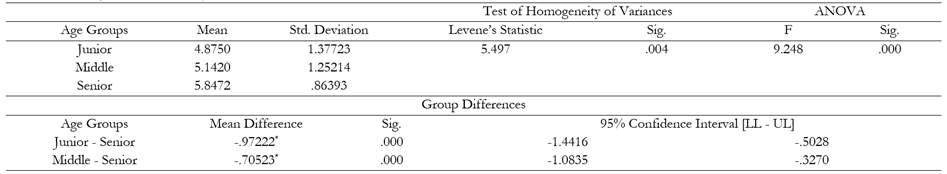To Learn How to Perform One Way ANOVA using SPSS, Please Watch the Video Tutorial